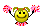## Recommended Posts

Hi! In my free time I have created little script that is simulating point moves in attractor energy field (http://en.wikipedia.org/wiki/Attractor ). At start you must input values of attractor field (i have alreade inputed some default values in script if you only want to test it few seconds) I think it is god fun, making some interesting attractorsp.s-sorry for my horrible englishsome screenshot:```#include <GUIConstants.au3>
\$guii=GUICreate("SET ATR DATA",300,300,-1,-1)
GUISetBkColor("0x666666",\$guii)
\$OK=GUICtrlCreateButton("START SIMULATION",150,270,150,30)
GUICtrlCreateLabel("x1 (start x coordinate)=",10,30,150,20)
GUICtrlCreateLabel("x2 (start y coordinate)=",10,70,150,20)
GUICtrlCreateLabel("z1 (start horizontal energy)=",10,110,150,20)
GUICtrlCreateLabel("z2 (start vertical energy)=",10,150,150,20)
GUICtrlCreateLabel("field energy=",10,190,150,20)
\$1=GUICtrlCreateInput("490",160,30,50,20,\$ES_CENTER)
\$2=GUICtrlCreateInput("278",160,70,50,20,\$ES_CENTER)
\$3=GUICtrlCreateInput("-1",160,110,50,20,\$ES_CENTER)
\$4=GUICtrlCreateInput("-0.5",160,150,50,20,\$ES_CENTER)
\$5=GUICtrlCreateInput("390",160,190,50,20,\$ES_CENTER)
\$typ=GUICtrlCreateCombo("Attractor",10,220,100,30)
GUICtrlSetData(\$typ,"Repeler")
Global \$x1=490, \$x2=178, \$z1=0, \$z2=-0.2, \$f_pola=390
GUISetState(@SW_SHOW)
While 1
\$msg = GUIGetMsg()
If \$msg = \$OK Then ExitLoop
If \$msg = \$GUI_EVENT_CLOSE Then ExitLoop
Wend
if \$typ="Attractor" Then _attr()
if \$typ="Repeler" then _repel()

Func _attr()
\$gui=GUICreate("Attractor Simple Simulator (Not 100% real!)", 500, 500,-1,-1)
GUISetBkColor(0x000000,\$gui)
GUISetState(@SW_SHOW)
\$a=GuiCtrlCreateGraphic(240, 240, 10,10)
GUICtrlSetGraphic(\$a,\$GUI_GR_COLOR, 0xeeeeee,0xaaaaaa)
GUICtrlSetGraphic(-1,\$GUI_GR_ELLIPSE, 2,2, 8,8)
\$b=GuiCtrlCreateGraphic(\$x1, \$x2, 10,10)
GUICtrlSetBkColor(-1,0x999999)
\$bg=GuiCtrlCreateGraphic(0, 0, 500,500)
GUICtrlSetBkColor(-1,\$GUI_BKCOLOR_TRANSPARENT)
\$pole=GuiCtrlCreateGraphic(145, 150, 200,200)
GUICtrlSetColor(-1,0x662222)
GUICtrlSetGraphic(\$bg,\$GUI_GR_MOVE, \$x1,\$x2)
\$timer=TimerInit()
do
sleep(2)
\$msg = GUIGetMsg()
if abs(\$x1-245)<100 and abs(\$x2-245)<100 Then
if \$x1<245 then \$z1+=((100-Abs(\$x1-245))/\$f_pola)
if \$x1>245 then \$z1-=((100-Abs(\$x1-245))/\$f_pola)
if \$x2<245 then \$z2+=((100-Abs(\$x2-245))/\$f_pola)
if \$x2>245 then \$z2-=((100-Abs(\$x2-245))/\$f_pola)
;TrayTip("SILY:","X: "&Round(\$x1,1)&@CRLF&"Y: "&Round(\$x1,1)&@CRLF&"F:z1= "&Round(\$z1,1)&@CRLF&"F:z2= "&Round(\$z2,1)&@CRLF&"Czas:"&Round(TimerDiff(\$timer)/1000,0),2)
endif
\$x1=\$x1+\$z1
\$x2=\$x2+\$z2
GUICtrlSetPos(\$b,\$x1,\$x2)
\$y1=\$x1+\$z1
\$y2=\$x2+\$z2
if \$x1< 0 or \$x2<0 or \$x2>500 or \$x1>500 then
MsgBox(0,"ZAKOŃCZONO/"&\$typ&" ENDED","Time in field: "&round(TimerDiff(\$timer)/1000,0)&" sec.")
exit(0)
endif
if abs(\$x1-245)<95 and abs(\$x2-245)<95 Then
GUICtrlSetGraphic(\$bg,\$GUI_GR_COLOR, 0x00ff00)
Else
GUICtrlSetGraphic(\$bg,\$GUI_GR_COLOR, 0xBBBB11)
endif
GUICtrlSetGraphic(\$bg,\$GUI_GR_LINE, \$y1+5,\$y2+5)
until \$msg = \$GUI_EVENT_CLOSE
EndFunc

Func _repel()
\$gui=GUICreate("Repeler Simple Simulator (Not 100% real!)", 500, 500,-1,-1)
GUISetBkColor(0x000000,\$gui)
GUISetState(@SW_SHOW)
\$a=GuiCtrlCreateGraphic(240, 240, 10,10)
GUICtrlSetGraphic(\$a,\$GUI_GR_COLOR, 0xeeeeee,0xaaaaaa)
GUICtrlSetGraphic(-1,\$GUI_GR_ELLIPSE, 2,2, 8,8)
\$b=GuiCtrlCreateGraphic(\$x1, \$x2, 10,10)
GUICtrlSetBkColor(-1,0x999999)
\$bg=GuiCtrlCreateGraphic(0, 0, 500,500)
GUICtrlSetBkColor(-1,\$GUI_BKCOLOR_TRANSPARENT)
\$pole=GuiCtrlCreateGraphic(145, 150, 200,200)
GUICtrlSetColor(-1,0x662222)
GUICtrlSetGraphic(\$bg,\$GUI_GR_MOVE, \$x1,\$x2)
\$timer=TimerInit()
do
sleep(2)
\$msg = GUIGetMsg()
if abs(\$x1-245)<100 and abs(\$x2-245)<100 Then
if \$x1<245 then \$z1-=((100-Abs(\$x1-245))/\$f_pola)
if \$x1>245 then \$z1+=((100-Abs(\$x1-245))/\$f_pola)
if \$x2<245 then \$z2-=((100-Abs(\$x2-245))/\$f_pola)
if \$x2>245 then \$z2+=((100-Abs(\$x2-245))/\$f_pola)
;TrayTip("SILY:","X: "&Round(\$x1,1)&@CRLF&"Y: "&Round(\$x1,1)&@CRLF&"F:z1= "&Round(\$z1,1)&@CRLF&"F:z2= "&Round(\$z2,1)&@CRLF&"Czas:"&Round(TimerDiff(\$timer)/1000,0),2)
endif
\$x1=\$x1+\$z1
\$x2=\$x2+\$z2
GUICtrlSetPos(\$b,\$x1,\$x2)
\$y1=\$x1+\$z1
\$y2=\$x2+\$z2
if \$x1< 0 or \$x2<0 or \$x2>500 or \$x1>500 then
MsgBox(0,"ZAKOŃCZONO/"&\$typ&" ENDED","Time in field: "&round(TimerDiff(\$timer)/1000,0)&" sec.")
exit(0)
endif
if abs(\$x1-245)<95 and abs(\$x2-245)<95 Then
GUICtrlSetGraphic(\$bg,\$GUI_GR_COLOR, 0x00ff00)
Else
GUICtrlSetGraphic(\$bg,\$GUI_GR_COLOR, 0xBBBB11)
endif
GUICtrlSetGraphic(\$bg,\$GUI_GR_LINE, \$y1+5,\$y2+5)
until \$msg = \$GUI_EVENT_CLOSE
EndFunc```
Edited by Uriziel01##### Share on other sites

Awesome!

P.S- your english is good!##### Share on other sites

Thank You for replyNow I'm writing segmental (LED) clockThis Attractor is only a test for more advanced graphical programs (I'm learning).

p.s-I have updated the first post with more recent sourceHave a good fun##### Share on other sites

Great idea with a nice output I'd say ;D good job!

##### Share on other sites

wow,

This looks good##### Share on other sites

As a student of science, I find this to be very cool.Throw in some nice OpenGL effects and a screensaver UDF and BAM you got yerself a very nice screensaver!

## Create an account

Register a new account

• ### Recently Browsing   0 members

×

• Wiki

• Back

• #### Beta

• Git
• FAQ
• Our Picks
×
• Create New...